# Problem 57417. Easy Sequences 86: The "real" Hyperprimorials

In Easy Sequences 85, we define the hyperprimorial of n, as a exponent ladder of primes numbers from the n-th prime down to first prime (2). OEIS, however, defines hyperprimorial differently. In OEIS sequence #A076265, the hyperprimorial of n, (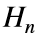) defined as follows:where: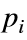is the i-th prime number.
Using the above formula, we want to calculate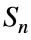, which is the sum of all hyperprimorials from 1 to n:For example: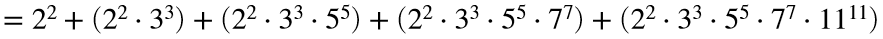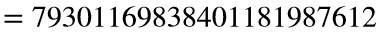.
Since this will involve large integers, please output a vector containing first 6, last 6, and the number of digits of. Therefore, the final function output should be: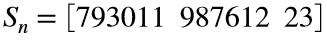.

### Solution Stats

100.0% Correct | 0.0% Incorrect
Last Solution submitted on Dec 18, 2022

### Community Treasure Hunt

Find the treasures in MATLAB Central and discover how the community can help you!

Start Hunting!